### Harbeck has Amnesia!

Wednesday, February 25, 2009
Harbeck fell and bumped his head.. he's like that guy, who bumped his head and couldn't get up till morning? Or something like that. Anyways, he found these weird things, and wants us to find out how they relate to each other, because he forgot! What did he find? Well, he found some weird looking shapes...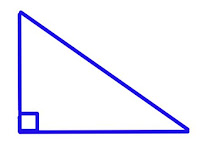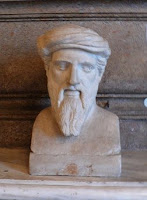He also found a weird looking formula! Also found a bust. Well I so happen to know what those shapes are, and that formula + the bust. But Harbeck had also found some words on a piece of paper.. legs, hypotenuse, R.A.T, Greek, theorem. I happen to know what those mean too! :) Sit back, relax and get ready to learn!Want to wonder what those words relate to the pictures? Well, then.. legs relate to the triangle. The legs make a big L or they can make up a 90 degree angle. If they can make up a right angle, they will be called complimentary angles! They will always be labeled as A or B.

The next word, hypotenuse is side C in the triangle. It is thelongest side, and is always located across the 90 degree angle.
R.A.T. stands for Right Angled Triangle, meaning, it has a right angle! (90 degree angle).

Greek relates to Pythagoras, the guy who's the bust. He was Greek and the father of math but we'll talk about that later..
Theorem? The Pythagorean Theorem is the theory that Pythagoras talks about A2 + B2= C2 (the 2's meaning squared).First, let's start off with a triangle. That triangle is called a right angled triangle, it only has one right angle. The right angle is made from the legs, or theta and beta. Theta and beta can also be called complimentary angles, because they make up a right angle as well.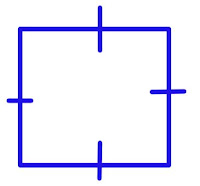Second is the square. A square has 4 equal sides, and to represent that the sides are equal, we draw lines on each 4 sides. There are 4 right angles in a square, and if you cut it from the corners, you can get a triangle!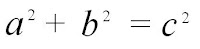The third picture is the Pythagorean theorem. The Pythagorean theorem explains how A2 is AxA + B2 is BxB. The sum of A2 and B2 equals to C2. I'm sorry if I can't explain this well, but it's easier said than done. My sister said this was sort of the way to explain it, so I will leave it like this.AND.. finally, the picture of Pythagoras' bust. He was a great, Greek mathematician. He made important developments in the theory of music, astronomy and math. Pythagoras usually hung out in Egypt and was a vegan. He was the first one to believe that the Earth was round, and that the Earth revolved around the sun.

Here is an example on how to solve the Pythagorean theorem.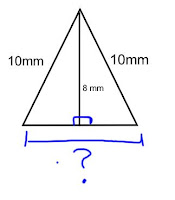(2 means squared. I can't find any other small 2 -_-)

A2+B2= C2
Let's label the 10mm as C, since it's the longest side.

Then, label the 8mm as A, or B. The bottom, A or B.

A2+8(2)= 10(2)-8(2) .. I put brackets so you won't get confused.
-8(2)

B2= 10(2)-8(2)
B2= (10x10) - (8x8)
B2= 100-64
B2= 36
(square root for both of them)
B= 6.
So, when you have only B or only A, you have to add the opposite. For example, 8(2)'s opposite would be -8(2).Another example of the Pythagorean theorem is finding the perimeter from finding the sides of the squares. Next, you will know what the sides A and B will be, because they're equal. After you have found A and B, you will have to find out what the hypotenuse is. After you've found out what the hypotenuse is, add up all the sides on the triangle to find out the perimeter of the game board!

Here are the two videos that Tracy, Peachy and Gelli made. I'm not in the video because I had an ortho, and now my teeth hurt -_- Well anyways, good job making the video, you guys!

Here you go Harbeck & .. classmates! :) I fail to explain things well, so I'm sorry if this is confusing. Like I said, easier said than done.